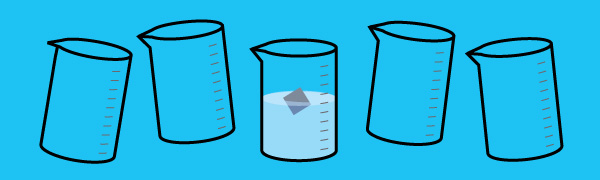# A Hot Floating CubeA metallic cube is floating inside a beaker. The temperature of the system is increased by a small amount ΔT. It is found that the depth of the submerged portion of the cube does not change. Then the ratio of the coefficient of linear expansion of the cube and the coefficient of volume expansion of the liquid is given by $\frac{a}{b},$ where $a$ and $b$ are coprime positive integers.

What is $a+b?$

Note: Ignore the expansion of the beaker.

×